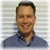## David’s Cisco Networking Blog

Aug 21 2008   8:29PM GMT

# Determining the Network ID using the Logical AND OperationProfile: David Davis

Studying for your CCNA? Having trouble with IP Subnetting?

It’s all about the 1’s and 0’s. We just need to convert our IP address and Subnet mask into binary and then subtract the ones from the zeros. 1+1 = 1, 1+0 = 0, 0+1 = 0. It really is that easy.

Let’s find out what the network ID is for this example.

The binary equivalent for IP address 140.12.26.128:
140 decimal is 10001100
12 decimal is    00001100
26 decimal is    00011010
128 decimal is 10000000
The binary for this IP address is:  10001100.00001100.00011010.10000000

Now let’s look at the binary equivalent for the IP subnet mask 255.255.240.0:
255 decimal is 11111111
255 decimal is 11111111
240 decimal is 11110000
0 decimal is      00000000
The binary for the subnet mask is:  11111111. 11111111. 11110000. 00000000
You have two steps left to complete. Subtract the binary IP address from the binary subnet mask
And then convert it to decimal.

Binary subnet mask:     11111111. 11111111. 11110000.00000000
====================================
Binary Result                  10001100.00001100.00010000.00000000
Decimal result:                    140.             12.            16.              0